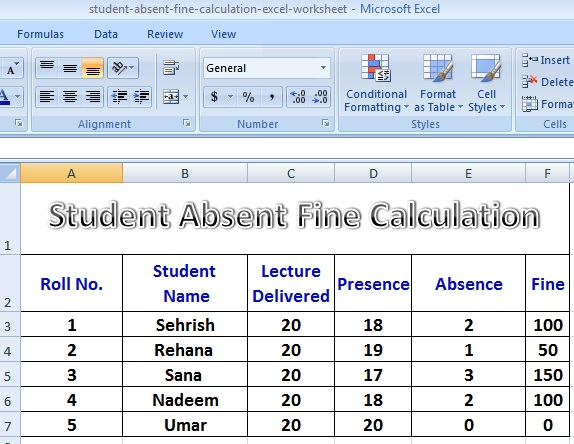# Excel Worksheet Student Absence Fine Calculation Formulas

By | April 11, 2018
•
•
•
•
•
•
•
•
•
•
Today, we will discuss how to create an Excel Worksheet that helps calculate Students Absence Fine Calculation on basis of Total Lectures Delivered and Total Lectures Attended (Presence) of the student.

### Steps To Prepare an Excel Worksheet for Student Absence Fine Calculation

1. Start MS Excel with a blank workbook option.
2. Type sample data in first Excel worksheet as shown in the given image.
3. Type formula for calculation of number of absence of a student.
Place cursor in Cell with reference E3.